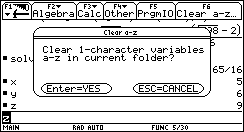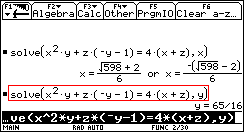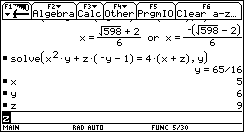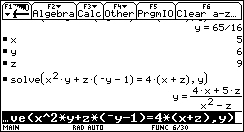IDEA: clear a-z cmd
03-21-2017, 03:01 PM (This post was last modified: 03-22-2017 09:31 PM by compsystems.)
Post: #1compsystemsSenior Member Posts: 1,343 Joined: Dec 2013
IDEA: clear a-z cmd
Hello sorry for my bad english

One of the problems for students, when a calculator as HP-PRIME or TI-89 stores a-z user variables, is that if one does not delete the variables, the results are evaluated, without showing the general or symbolic solutions.

See publication in the following link http://courses.wccnet.edu/~palay/math179/intslv92.htm

The TI68k have an option to remove the symbolic variables a-z, I think it would be a good option to incorporate it into the HP-PRIME for cas MODE, The RESTART CMD works but deletes all definitions =(, then a single command is required for the alphabetic variables, or expand RESTART CMD, accepting arguments RESTART([arg])

RESTART( "ALL" );
RESTART( "a-z" );CAS VIEW
PHP Code:
CAS VIEWCASE 1:  Preassigning values for x,y,z lowercasex:=5; y:=6; z:=9;//1.1: solve lowercase & Maximum simplifysolve( x^2*y+z*(-y-1) = 4*(x+z), x ); // returns { (-√(598)+2)/6,  (√(598)+2)/6 }  // OK, As x,y,z have values, solve cmd solve then evaluateapprox(Ans); {  −3.742 , 4.409 }  // -> x=4.40 or x=−3.74 // ok//1.2: SOLVE uppercaseSOLVE( x^2*y+z*(-y-1) = 4*(x+z), x );  orSOLVE( x^2*y+z*(-y-1) = 4*(x+z), x, 6 );// returns 4.40900642021 SOLVE( x^2*y+z*(-y-1) = 4*(x+z), x, -6 );// returns −3.742 CASE 2: Deleting variables x,y,zpurge(x,y,z); //2.1: solve lowercasesolve( x^2*y+z*(-y-1) = 4*(x+z), x );  // returns { (√(y^2*z+5*y*z+4)+2)/y,   (-√(y^2*z+5*y*z+4)+2)/y } // Symbolic result for X1/2{ (√(y^2*z+5*y*z+4)+2)/y, (-(√(y^2*z+5*y*z+4))+2)/y}|({y = 6,z = 9}); returns{  4.409, −3.742  }solve( x^2*y+z*(-y-1) = 4*(x+z), y );  // returns {(4*x+5*z)/(x^2-z)}// Symbolic result for Y{(4*x+5*z)/(x^2-z)}|({x = 5,z = 9}); returns {65/16}//2.2: SOLVE uppercaseSOLVE( x^2*y+z*(-y-1) = 4*(x+z), x, 6 );  returns "Error: Bad Guess(es)" SOLVE( x^2*y+z*(-y-1) = 4*(x+z), x, -6 ); returns "Error: Bad Guess(es)"SOLVE( x^2*y+z*(-y-1) = 4*(x+z), y, -6 ); returns "Error: Bad Guess(es)"CASE 3:  Non-zero values for X uppercaseX:=5; Y:=6; Z:=9;//3.1: solve lowercase  solve( X^2*Y+Z*(-Y-1) = 4*(X+Z), X); // returns { (-√(598)+2)/6,  (√(598)+2)/6 }  // OK, As x,y,z have values, solve cmd solve then evaluateapprox(Ans); {  −3.742 , 4.409 }  // -> x=4.40 or x=−3.74 // ok//3.2: SOLVE uppercaseSOLVE( X^2*Y+Z*(-Y-1) = 4*(X+Z), X);  orSOLVE( X^2*Y+Z*(-Y-1) = 4*(X+Z), X, 6); // returns 4.40900642021  SOLVE( X^2*Y+Z*(-Y-1) = 4*(X+Z), X, -6);// returns −3.742  CASE 4: Restting variables x,y,zX:=0; Y:=0; Z:=0; //4.1: solve lowercasesolve( X^2*Y+Z*(-Y-1) = 4*(X+Z), X); // returns { 0 }//4.2: SOLVE uppercaseSOLVE( X^2*Y+Z*(-Y-1) = 4*(X+Z), X, 6);  returns0

HOME VIEW
PHP Code:
HOME VIEWSOLVE(Eq, var, guess) // SOLVE CMD for HOMECASE 5:X:=5; Y:=6; Z:=9;SOLVE( X^2*Y+Z*(-Y-1) = 4*(X+Z), X); returns 4.40900642021 solve( X^2*Y+Z*(-Y-1) = 4*(X+Z), X);  returns {X}CASE 6: Restting variables x, y, zX:=0; Y:=0; Z:=0; SOLVE( X^2*Y+Z*(-Y-1) = 4*(X+Z), X); returns 4.40900642021solve( X^2*Y+Z*(-Y-1) = 4*(X+Z), X); returns {X}
03-21-2017, 08:33 PM
Post: #2
 Arno KSenior Member Posts: 469 Joined: Mar 2015
RE: IDEA: clear a-z cmd
Is √(y^2*z+5*y*z+4)+2)/y different from √(598)+2)/6) with y=6 and z=9? No, so the result is correct.
So one has to be aware of the variables one has used before and then gets the desired symbolic results. I myself usually nearly never assign values to variables used in cas and have some rules I follow when I do so. Nevertheless the idea of spending purge some more parameters is not bad.
Arno
03-22-2017, 05:48 AM
Post: #3
 cyrille de brébissonSenior Member Posts: 1,047 Joined: Dec 2013
RE: IDEA: clear a-z cmd
Hello,

One of the issue here is the meaning of the word "variable".

In symbolic math, a variable is a name used in replacement of an unknown value. In the symbolic word, a variable does not have any value (at least most of the time).

In lower grade math, in "computational" math, in physics or in programming worlds, a variable is a named container for a value. a Variable always has a value which it temporary replaces for clarity, readability or to allow a series of final computations with various values for the said variables.

Prime does have both these worlds in it. Home is definitely computational math. Cas is symbolic. But the 2 also interact to add to the confusionThe end result is Prime. Power and flexibility, but at the cost of the need to understand such subtility.

In your case, remember that by default, A-Z variables always have a value (computational) and a-z (lowercase) do not (symbolic). And you should be OK.

Hope this helps.

Cyrille

Although I work for the HP calculator group, the views and opinions I post here are my own. I do not speak for HP.
03-22-2017, 03:03 PM
Post: #4KeithBSenior Member Posts: 409 Joined: Jan 2017
RE: IDEA: clear a-z cmd
That is fine for FORTRAN programmers that can use I for a loop variable, but what about us C programmers that have to use i? 8^)

I suggest that the OP write his own darn function/program if it is that important to him.
03-22-2017, 06:42 PM (This post was last modified: 03-22-2017 09:30 PM by compsystems.)
Post: #5compsystemsSenior Member Posts: 1,343 Joined: Dec 2013
RE: IDEA: clear a-z cmd
The SOLVE in capital letters is not documented in the CATALOG HELP

Quote:solve
Returns a list of the solutions (real and complex) to a polynomial equation or a set of polynomial equations.

solve(Eq,[Var]) or
solve({Eq1, Eq2,…}, [Var]) or
solve(Eq, Var=Guess) or
solve(Eq, Var=Val1…Val2)

Quote:SOLVE
Solves an equation for one of its variables. Solves the equation En for the variable var, using the value of guess as the initial value for the value of the variable var. If En is an expression, then the value of the variable var that makes the expression equal to zero is returned.

SOLVE(En,var,guess)

This function also returns an integer that is indicative of the type of solution found, as follows:
0—an exact solution was found
1—an approximate solution was found
2—an extremum was found that is as close to a solution as possible
3—neither a solution, an approximation, nor an extremum was found

I do not understand the last part [0 - 3]

Update the examples of the first post, I think that THE DIFFERENT CASES should have a more detailed explanation by Bernard
03-23-2017, 04:52 PM
Post: #6
 parisseSenior Member Posts: 1,301 Joined: Dec 2013
RE: IDEA: clear a-z cmd
solve(equation) solves equation with respect to x
solve(equation,var) solves equation with respect to var
solve([eq1,eq2,..],[var1,var2,...]) solve polynomial system with respect to var1,var2,...
The last syntaxes call the numeric solver, using an iterative method (var=guess) or bisection (var=a..b).
03-24-2017, 08:21 PM
Post: #7compsystemsSenior Member Posts: 1,343 Joined: Dec 2013
RE: IDEA: clear a-z cmd
SOLVE CMD in capitals should not evaluate after solving, when the variables are also capitals, because by default X, Y, ... are initialized to zero and there is no way to eliminate previously.